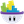#### Howdy, Stranger!

It looks like you're new here. If you want to get involved, click one of these buttons!

# identifying actors with x/y coordinates...

edited November -1
what's cookin,

is there a way to check an x/y position and ask if an actor is at that current position? any part of the actor, not just the center position.

i want to be able know if an actor is in a particular area without having to collide with that actor to identify it.

something like: if ((pos x)(pos y))==('enemy' actor) then ...

• Jah, create two real attributes with the values of where you want to see if your actor's at. Then create a rule that says, if self.positionX = AttributeX and self.positionY = AttributeY, etc...
• Posts: 1,803Head Chef, Member, PROWell if it is only one point(x',y') that you want to check if an actor has hit it, then its pretty easy.

Rectangular collision actor: (self.x-width/2<=x'<=self.x+width/2)
if attribute changes self.position.X <= x'+self.Size.width/2
and
if attribute changes self.position.X >= x'-self.Size.width/2
and
if attribute changes self.position.Y <= y'+self.Size.height/2
and
if attribute changes self.position.Y >= y'-self.Size.height/2

Note: Since there is no greater than or equal to , nor less than or equal to, each of the conditions above needs to be 2 conditions. I'll check into adding >= and <=.

Circular collisions: distance between the 2 points is less than or equal to the radius of the circle.
if attribute changes, self.width (or height, whichever is smaller and thus is the radius) >= 2*magnitude(self.Position.X-x', self.Position.Y-y')
• what's cookin,

kamazar: i started down that path of reasoning but i think, and correct me chefs if i'm wrong but, the position variables only reference the center point of the actor. i want to be able to just determine an x/y point and see if there is an actor at that point, even if it is just a corner piece of the actor touching that particular x/y point.

codemonkey: i thought that i might be able to use an invisible, collidable, 1 pixel actor to see if any other actor is at that point. but then i thought, for memory usage and optimum speed, it would be better if there were a behavior,method or reference where i could just input the x/y parameter and return if an actor currently resided at that point.
please let me know if i'm interpreting this wrong but it looks like your method is seeing if a generic x/y point is contained within the height and width of a specific actor. i want to go somewhat the opposite way and have a generic point that i define and discover if there is any actor currently intersecting that point. that way, i won't have to deal with the dimensions of any specific actor, i can just see if any actor is on this point at any time.

is this doable?

i'll draw up a quick diagram illustrating why i want this, as opposed to using invisible collidable actors.
• what's cookin,

here's the diagram of what i'm trying to accomplish.